Home » SQL Server Sequence

# SQL Server Sequence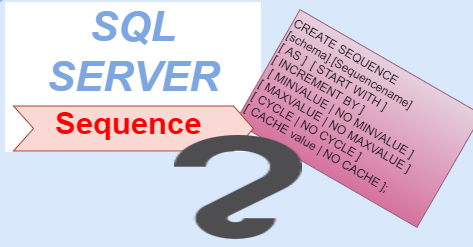SQL Server Sequence objects are used to sequentially generate numeric values that can be in ascending or descending order at a defined interval and may cycle if requested.

Sequence objects are similar to the IDENTITY column in any SQL table. However, unlike the IDENTITY column, they are independent and are not attached to any table.

Sequence objects are used both independently and within the DML statements that is INSERT, UPDATE and DELETE.

SYNTAX

```CREATE SEQUENCE [schema].[Sequencename]

[ AS <data type> ]

[ INCREMENT BY <value> ]

[ MINVALUE <value > | NO MINVALUE ]

[ MAXVALUE <value> | NO MAXVALUE ]

[ CYCLE | NO CYCLE ]

[ CACHE value | NO CACHE ];```

CREATE SEQUENCE  Used to create a sequence followed by a database schema and the name of the sequence.

AS is the default value for the data type is BigInt, data types can be Int, SmallInt, TinyInt, BigInt and Decimal, .

INCREMENT BY it can be either a positive or negative value. If a positive value is specified, the sequence will be an ascending sequence of values. If a negative value is specified, the sequence will be a descending sequence of values.

MINVALUE  is the minimum value for the sequence object.

NO MINVALUE  means that no minimum value specified for the sequence object.

MAXVALUE  is an optional parameter that sets the maximum value for the sequence object.

NO MAXVALUE  means that no maximum value specified for the sequence object.

CYCLE  sequence will start over once it has completed the sequence.

NO CYCLE  It will not start the sequence over again.

CACHE is used to cache sequence object values.

NO CACHE  It does not cache the sequence Objects.

Lets look at an example of Sequences in SQL.

#### Creating a Sequence

Following Statement create a sequence object named GenSeqId. The type of this sequence object is integer.

Sequence Objects starts from 100 and increments by 2.

```CREATE SEQUENCE dbo.GenSeqId
AS INT
INCREMENT BY 2```

You can check the details of a sequence using following statement.

```SELECT *
FROM sys.sequences
WHERE name = 'GenSeqId';```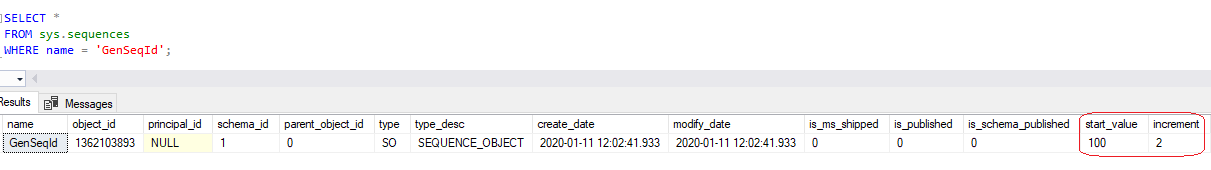To see the value that GenSeqId sequence object contains , use the following statement.

`SELECT NEXT VALUE FOR [dbo].[GenSeqId]`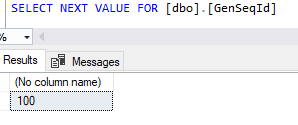```Create table EmpData(
EmpId int ,
EmpName varchar(100)
)```

#### Using a Sequences with Insert statement

Lets create a table EmpData.
```Create table EmpData

( EmpId int,

EmpName varchar(50)

)```
Now, we insert some records in the EmpData table, For the EmpId column of the table we use the following statement
```INSERT INTO EmpData VALUES ( NEXT VALUE FOR [dbo].[GenSeqId],'NICK ANTHONY')

INSERT INTO EmpData VALUES ( NEXT VALUE FOR [dbo].[GenSeqId],'JACK MARK A')```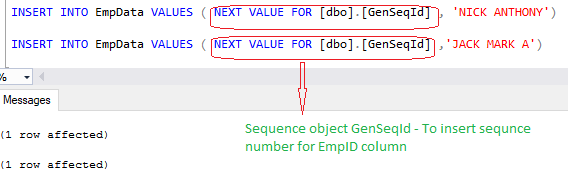Lets select the records, as you can see the output that the value for EmpId column is generated by the GenSeqId sequence  object that is 102 and 104 .

`SELECT * FROM EmpData`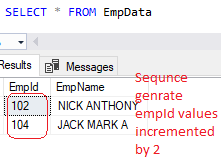#### Modifying a sequence objects

To modify an existing sequence, the ALTER SEQUENCE statement is used.

The following statement modifies an existing sequence object ‘GenSeqId’ by updating its starting value to 100

```ALTER SEQUENCE [GenSeqId]
```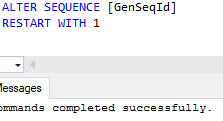Lets check the value that GenSeqId sequence object holds now , as you can see it is 1, that means sequence objects value is updated.
`SELECT NEXT VALUE FOR [dbo].[GenSeqId]`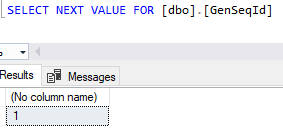#### Sequence with Max and Min Values

Lets alter the GenSeqNumber and set min and Max values for it.

```CREATE SEQUENCE [dbo].[GenSeqNumber]
AS INT
INCREMENT BY 2
MINVALUE 1
MAXVALUE 6```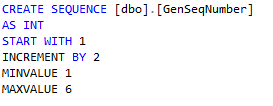As you can see above, we have created a sequence object named ‘GenSeqNumber‘, and it has a starting value that is incremented by 2 and the minimum value for this sequence object is 1 and maximum value is 6.

Now if you increment the value of this sequence object beyond 6, It returns an error.

As the maximum value for sequence object is set to 6, so when sequence object is executed for 3 time the value will be exceeding the maximum value 6 then it gives an error.

Lets execute the  below statement for 3 three times and you can see it gives an error.

`Select NEXT VALUE FOR [dbo].[GenSeqNumber]`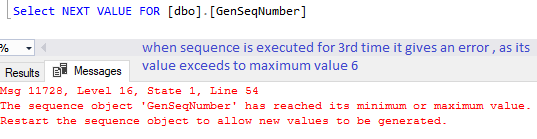#### Sequence with CYCLE

As you see above, when increment value of a sequence object go beyond to its maximum value, it gives an error, in case this case to avoid error , you can use a CYCLE flag.

If a CYCLE flag for a sequence is set to true, the value for the sequence object is again set to its starting value.

```CREATE SEQUENCE [dbo].[GenSeqNumber]

AS INT

INCREMENT BY 2

MINVALUE 1

MAXVALUE 6

CYCLE```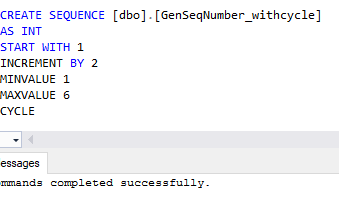When Sequence objected is executed and exceeds it’s maximum value the sequence value is reset to start value again.

```SELECT NEXT VALUE FOR [dbo].[GenSeqNumber_withcycle]
SELECT NEXT VALUE FOR [dbo].[GenSeqNumber_withcycle]
SELECT NEXT VALUE FOR [dbo].[GenSeqNumber_withcycle]```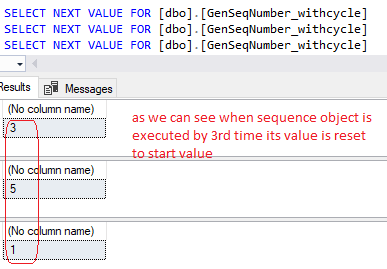#### Drop Sequence

To drop a sequence objects, you can use drop statement as given below.

`Drop sequence [GenSeqNumber_withcycle]`